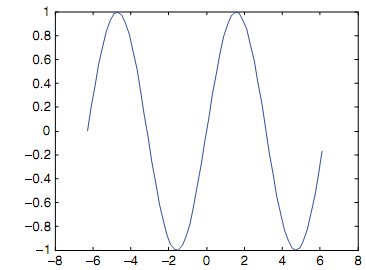## Example to change the line width from the default, MATLAB in Engineering

Assignment Help:

Example to change the line width from the default:

For illustration, to change the line width from the default of 0.5 to 1.5:

>> set(hl,'LineWidth',1.5)

As long as the Figure Window is open and this object handle is still valid, the width of the line will be increased.

Also the properties can be set in the original function call. For illustration, this will increase the line width as shown in figure.

>> hl = plot(x,y, 'Linewidth', 2.5);#### For loop, FOR Loop: The for loop, or the for statement, is used whenev...

FOR Loop: The for loop, or the for statement, is used whenever it is essential to repeat statement(s) in the script or function, and whenever it is known ahead of time how man

#### Algorithm for appex subfunction, Algorithm for appex subfunction: The ...

Algorithm for appex subfunction: The algorithm for appex subfunction is as shown:  Receives x & n as the input arguments.  Initializes a variable for running sum of t

#### Smoothing values, How can I use the weighted moving average formula in matl...

How can I use the weighted moving average formula in matlab to smooth a column data of 404 values?

#### Example of plotting from a function, Example of Plotting from a Function: ...

Example of Plotting from a Function: For illustration, the function can be called as shown below:      >> y = [1:2:9].^3      y =     1  27  125  343  729

#### Function numden, function numden: The function numden will return indi...

function numden: The function numden will return individually the numerator & denominator of a symbolic expression: >> sym(1/3 + 1/2) ans = 5/6 >> [n, d] =

#### Plotting from a function, Plotting from a Function: The following func...

Plotting from a Function: The following function creates a Figure Window as shown in figure, which shows various types of plots for similar y vector. The vector is passed as a

#### Example of modular program, Example of modular program: In a modular p...

Example of modular program: In a modular program, there would be one main script which calls three separate functions to complete these tasks: A function to prompt an us

#### Reduced row echelon form, Reduced Row Echelon Form: The Gauss Jordan t...

Reduced Row Echelon Form: The Gauss Jordan technique results in a diagonal form; for illustration, for a 3 × 3 system: The Reduced Row Echelon Forms take this one step

#### Structures, Structures: The Structures are data structures which group...

Structures: The Structures are data structures which group together values which are logically related in what are known as the fields of structure. The benefit of structures

#### Function used in sound files, Function used in sound files: The MATLAB...

Function used in sound files: The MATLAB has numerous other functions which let you read and play sound or audio files. In the audio files, sampled data for each audio channel